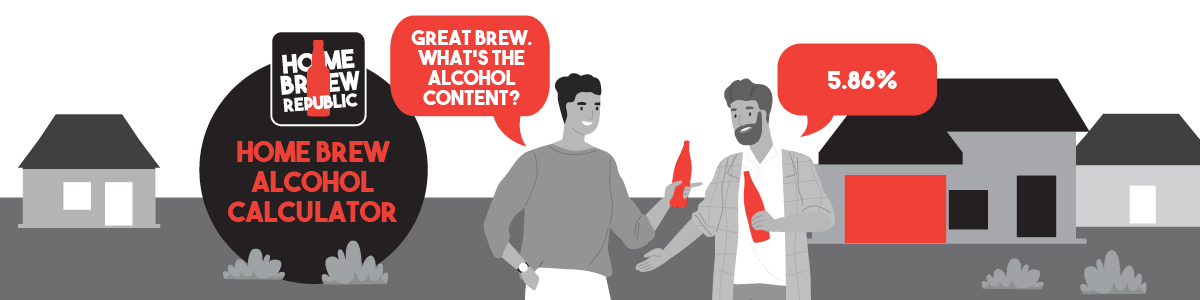## Home Brew Republic Homebrew Alcohol Calclator | Home Brew Beer ABV Calculator

### The Home Brew Republic Specific Gravity ABV Calculator for Home Brew Beer and Cider.

The Homebrew Alcohol Calculator makes it easy for you to calculate the alcohol by volume for your latest brew. This specific gravity ABV calculator can be used for beer or cider.

Simply enter your Original Gravity (OG) reading, which you took before fermentation commenced and your Final Gravity (FG) reading, which you took when fermentation concluded and the and the specific gravity ABV calculator will give you the estimated alcohol content for your brew.

Note: Please do not use this homebrew alcohol calculator for wine.

Original Gravity (OG):

Final Gravity (FG):

At this point we’re thinking a small disclaimer might be appropriate for the Home Brew Republic Alcohol Calculator. The ABV calculated by the Homebrew Alcohol Calculator should be treated as an approximate estimate for the alcohol content of beer and cider that is home brewed. Accuracy of the ABV provided from the specific gravity calculator is dependent on the Original and Final Gravity readings taken by you and the temperature they were taken at.

## How the Homebrew Alcohol Calculator Works

### What is alcohol by volume (ABV)?

Alcohol by volume is standard unit of measurement of how much alcohol (ethonol) is contained in a given volume of an alcoholic beverage. It is expressed as a percentage of the total volume, meaning this is the number of millilitres (ml) of pure ethanol present per 100 ml of a beverage.

You can easily calculate the estimated ABV of your home brews using the homebrew alcohol calculator or you can perform the calculation manually.

### How is ABV calculated?

The Home Brew Republic specific gravity calculator uses a universal formula to calculate the estimated ABV of your brew.

#### (OG – FG) x 131.25

OG being Original Gravity and FG being Final Gravity.

For example if my OG was 1.042 and my FG was 1.008 then:

1.042 – 1.008 = 0.34

0.34 – 131.25 = 4.46

My estimated ABV in this example would be 4.46%

### Fermentation, gravity and ABV in a little more detail.

To create alcohol in our brews, yeast convert sugars and other fermentable materials in our wort during the process of fermentation.

To estimate ABV we use the gravity of our beer or cider. Gravity refers to the density of the solution. In wort we are dealing with two items contributing to the overall density of the solution. The first is water which makes up greater than 90% of the solution and the second is sugar which is added.

Sugar is a larger and heavier molecule than water. When sugar is dissolved in water, the density of the water is increased. In the finished beer or cider, some of the sugars and fermentable materials are converted to alcohol which lowers the density of the solution. Original Gravity (OG) refers to the density of the wort before fermentation and Final Gravity (FG) refers to the density of the wort post fermentation. We use the difference in gravity to determine how much alcohol there is now in our solution.

If you’re wondering why brewers use 131.25 as the multiplier in the ABV calculation (we did), the number comes from a chemistry equation that includes the density of ethanol and the number of ethanol grams generated per gram of dioxide released. Brewers have been using this same formula for centuries.